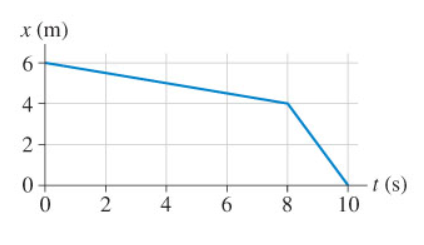# Problem: The position graph of the figure shows a dog slowly sneaking up on a squirrel, then putting on a burst of speed.For how many seconds does the dog move at the slower speed? (1 sigfig with appropriate units)

###### FREE Expert Solution

The slope of the position-time graph gives the velocity.

There are two parts of motion in the graph, between times 0s and 8 s, and between times 8 s and 10 s.###### Problem DetailsThe position graph of the figure shows a dog slowly sneaking up on a squirrel, then putting on a burst of speed.
For how many seconds does the dog move at the slower speed? (1 sigfig with appropriate units)